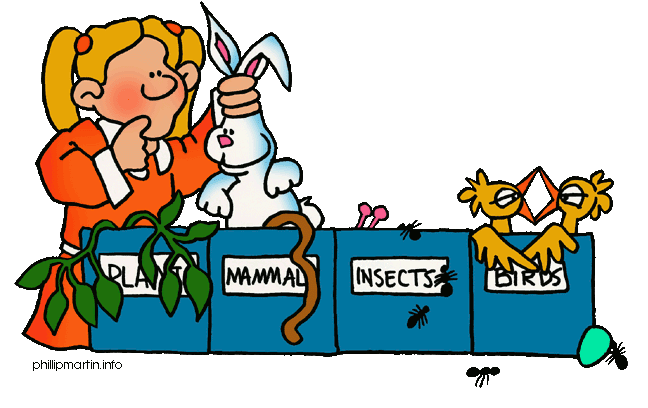# 朴素贝叶斯分类器的应用症状　　职业　　　疾病

打喷嚏　护士　　　感冒
打喷嚏　农夫　　　过敏
头痛　　建筑工人　脑震荡
头痛　　建筑工人　感冒
打喷嚏　教师　　　感冒
头痛　　教师　　　脑震荡

P(A|B) = P(B|A) P(A) / P(B)

P(感冒|打喷嚏x建筑工人)
= P(打喷嚏x建筑工人|感冒) x P(感冒)
/ P(打喷嚏x建筑工人)

P(感冒|打喷嚏x建筑工人)
= P(打喷嚏|感冒) x P(建筑工人|感冒) x P(感冒)
/ P(打喷嚏) x P(建筑工人)

P(感冒|打喷嚏x建筑工人)
= 0.66 x 0.33 x 0.5 / 0.5 x 0.33
= 0.66

P(C|F1F2...Fn)
= P(F1F2...Fn|C)P(C) / P(F1F2...Fn)

P(F1F2...Fn|C)P(C)

P(F1F2...Fn|C)P(C)
= P(F1|C)P(F2|C) ... P(Fn|C)P(C)

C0 = 0.89

C1 = 0.11

F1: 日志数量/注册天数
F2: 好友数量/注册天数
F3: 是否使用真实头像（真实头像为1，非真实头像为0）

F1 = 0.1
F2 = 0.2
F3 = 0

P(F1|C)P(F2|C)P(F3|C)P(C)

P(F1|C0) = 0.5, P(F1|C1) = 0.1
P(F2|C0) = 0.7, P(F2|C1) = 0.2
P(F3|C0) = 0.2, P(F3|C1) = 0.9

P(F1|C0) P(F2|C0) P(F3|C0) P(C0)
= 0.5 x 0.7 x 0.2 x 0.89
= 0.0623

P(F1|C1) P(F2|C1) P(F3|C1) P(C1)
= 0.1 x 0.2 x 0.9 x 0.11
= 0.00198

性别　　身高（英尺）　体重（磅）　　脚掌（英寸）

男 　　　6 　　　　　　180　　　　　12
男 　　　5.92　　　　　190　　　　　11
男 　　　5.58　　　　　170　　　　　12
男 　　　5.92　　　　　165　　　　　10
女 　　　5 　　　　　　100　　　　　6
女 　　　5.5 　　　　　150　　　　　8
女 　　　5.42　　　　　130　　　　　7
女 　　　5.75　　　　　150　　　　　9

P(身高|性别) x P(体重|性别) x P(脚掌|性别) x P(性别)P(身高=6|男) x P(体重=130|男) x P(脚掌=8|男) x P(男)
= 6.1984 x e-9

P(身高=6|女) x P(体重=130|女) x P(脚掌=8|女) x P(女)
= 5.3778 x e-4

（完）

## 留言（67条）

……/ { P(打喷嚏) x P(建筑工人) }

Thank you very much for sharing!!!

thanks a lot, 让我对数学又恢复了激情

0.66 x 0.33 x 0.5 / 0.5 x 0.33

@不告诉你：

“所以，男性的身高为6英尺的概率等于1.5789（大于1并没有关系，因为这里是密度函数的值）”——我理解是不是因为最终只是比较相对大小，做一个判定，所以直接采用密度函数的值作为概率值？因为理论上连续变量取某一个具体值的概率都是无穷小。

@刑无刀：

`引用zhangxaochen的发言：`

`引用Kyle的发言：`

1.5789 是怎么算出来的呢？ 我算得 0.002137402507386169

C# 代码 正态 分布计算：

using System;
using System.Collections.Generic;
using System.Linq;
using System.Text;

namespace ConsoleApplication1
{
class Program
{
static void Main(string[] args)
{
Console.WriteLine(NormalDist(6, 5.855, 0.035));
}
//第一个参数是 你的数据，第二个是平均值，第三个是方差
public static double NormalDist(double x, double mean, double standard_dev)
{
double fact = standard_dev * Math.Sqrt(2.0 * Math.PI);
double expo = (x - mean) * (x - mean) / (2.0 * standard_dev * standard_dev);
return Math.Exp(-expo) / fact;
}
}
}

`引用阿宝的发言：`

1.5789 是怎么算出来的呢？ 我算得 0.002137402507386169

P(感冒|打喷嚏x建筑工人)
= P(打喷嚏|感冒) x P(建筑工人|感冒) x P(感冒)
/ P(打喷嚏) x P(建筑工人)

`引用李瑞的发言：`

P(感冒|打喷嚏x建筑工人)
= P(打喷嚏|感冒) x P(建筑工人|感冒) x P(感冒)
/ P(打喷嚏) x P(建筑工人)

P(打喷嚏x建筑工人)=P(打喷嚏) x P(建筑工人)

P(打喷嚏x建筑工人|感冒) =P(打喷嚏|感冒) x P(建筑工人|感冒)

0.035 怎么计算来的？

`引用不告诉你的发言：`

0.66 x 0.33 x 0.5 / 0.5 x 0.33

`引用不告诉你的发言：`

0.66 x 0.33 x 0.5 / 0.5 x 0.33

P(A|B) 的意思是，在 B 发生的前提下，A 出现的几率是多少。

P(身高=6|男) x P(体重=130|男) x P(脚掌=8|男) x P(男)
= 6.1984 x e-9

P(身高=6|男) = 1.5789
P(体重=130|男) = 3.80208624997982E-7
P(脚掌=8|男) = 2.2187198416957028E-4
P(男) = 0.5

“所以，男性的身高为6英尺的概率等于1.5789（大于1并没有关系，因为这里是密度函数的值）”这里不大同意哦，学过概率的都知道，如果是正态分布的话，均值两边是对称的，均值是5的话，那么身高6和4的概率密度值是相同。难道这样还不影响最终结果么。所以我的建议是既然概率密度函数知道了，那么直接求概率（放到分布函数中）即可！！

`引用Lyon的发言：`

`引用maggieli的发言：`

`引用wfh的发言：`

P(打喷嚏)是0.66啊，怎么又成了0.5 ？

>>> 虽然"所有特征彼此独立"这个假设，在现实中不太可能成立，但是它可以大大简化计算，而且有研究表明对分类结果的准确性影响不大。

«-必填

«-必填，不公开

«-我信任你，不会填写广告链接